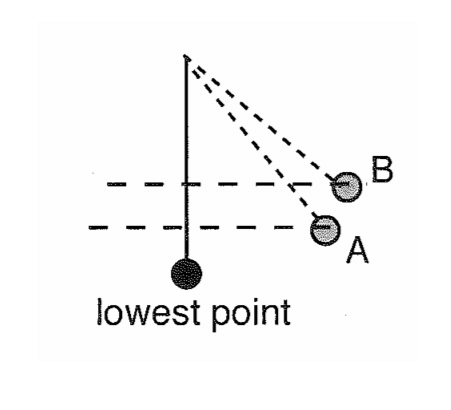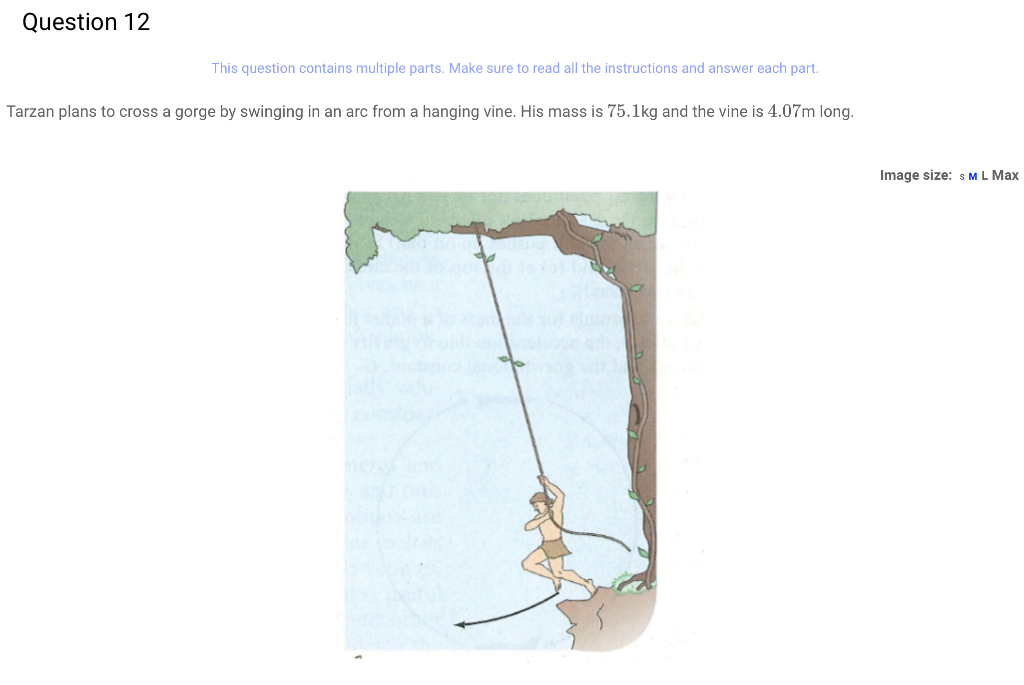you are traveling 6.8 m/s at the lowest point on the swing. your mass is 80kg. what is the heighdifference between the highest and lowest points of the swing?

V^2 = 2gh H = v^2/2g = (6.8)^2/2×9.8 = 2.359 m
source: Me
Similar Homework Help Questions
• ### force,tension

A child weighing 200 N is swinging back and forth on a rope attached to a tree branch. The rope is 3.7 m long and has negligible mass.(a) At the lowest point of the swing, the child is moving with speed 4.0 m/s. What is the tension in the rope at this instant?(b) What is the direction and magnitude of the child’s acceleration at the highest point of the swing when the rope makes an angle ? with the vertical?(c)...

• ### Need Help

1 A child weighing 220 N is swinging back and forth on a rope attached to a tree branch. Therope is 3.8 m long and has negligible mass.a) At the lowest point of the swing, the child is moving with speed 4.2 m/s. What is the tensionin the rope at this instant?b) What is the direction and magnitude of the child’s acceleration at the highest point of theswing when the rope makes an angle ? with the vertical?c) What is...

• ### A pendulum has 343 J of kinetic energy at the lowest point in its swing

A pendulum has 343 J of kinetic energy at the lowest point in its swing. At the highest point in its swing, it has a height of 5 m. What is the pendulum's mass?

• ### I found part a, but need help with part b: Tarzan plans to cross a gorge...

I found part a, but need help with part b: Tarzan plans to cross a gorge by swinging in an arc from a hanging vine. His mass is 83.58 kg and the vine is 3.01 m long. a. If his arms are capable of exerting a force of 14001400 N on the rope, what is the maximum speed he can tolerate at the lowest point of his swing? Answer: 4.58 m/s b. On the other side of the gorge, at...

• ### Work/Velocity

A 20 kg child on a playground swing moves with a speed of 3.8 m/s when the 6.0 m long swing is at its lowest point. What is the angle that the swing makes with thevertical when the child is at the highest point?

• ### conservation of energy #3

A 13 kg child on a playground swing moveswith a speed of 2.9 m/s when the 6.0 mlong swing is at its lowest point. What is the angle that the swingmakes with thevertical when the child is at the highest point?

• ### #66 GP

Tarzan wants to cross a gorge by swinging in an arc from a vertical vine. His arms are capable of exerting a 1400N force on the vine, what is the max. speed he cantolerate at the lowest point of his swing? His mass= 80kg and vine is 5.5m long.

• ### A 37.0-kg child swings in a swing supported by two chains, each 3.06 m long. The...

A 37.0-kg child swings in a swing supported by two chains, each 3.06 m long. The tension in each chain at the lowest point is 354 N. (a) Find the child's speed at the lowest point. 5.8 Incorrect: Your answer is incorrect. Can you find the net force on the child at the lowest point of the swing? Do you expect this to be zero if the child is moving in a circle? m/s (b) Find the force exerted by...

• ### A simple pendulum of mass 0.2 kg and length 0.4 m oscillates freely. At the end...A simple pendulum of mass 0.2 kg and length 0.4 m oscillates freely. At the end of its swing (point B), it has 0.45 J of gravitational potential energy with respect to its lowest point. Determine the total mechanical energy of the pendulum when it is halfway in height (point A) between its lowest point and the end of its swing. Show your calculations/explain your reasoning. В A lowest point

• ### Question 12 This question contains multiple parts. Make sure to read all the instructions and answer...Question 12 This question contains multiple parts. Make sure to read all the instructions and answer each part. Tarzan plans to cross a gorge by swinging in an arc from a hanging vine. His mass is 75.1kg and the vine is 4.07m long. Image size: s ML Max Part a (1 points) If his arms are capable of exerting a force of 1400 N on the rope, what is the maximum speed he can tolerate at the lowest point of...

Free Homework App